# vs.eyeandcontacts.com

## Chapter 13 Direct and Inverse Proportions Exercise 13.2

Question 1: Which of the following are in inverse proportion?
i) The number of workers on a job and the time to complete the job.
ii) The time taken for a journey and the distance travelled in a uniform speed.
iii) Area of cultivated land and the crop harvested.
iv) The time taken for a fixed journey and the speed of the vehicle.
v) The population of a country and the area of land per person.

i) Inverse proportion; If the workers are more, the time taken will be less. If the workers are less, the time taken will be more.
ii) Direct proportion; Time and distance are in direct proportion.
iii) Direction proportion; More the cultivated land more yield more crops.
iv) Inverse proportion; If the time is less, the speed will be more
v) Inverse proportion; If the population of a country increases, the area of land per person decreases.

Question 2: In a Television game show, the prize money of Rs 1,00,000 is to be divided equally amongst the winners. Complete the following table and find whether the prize money given to an individual winner is directly or inversely proportional to the number of winners?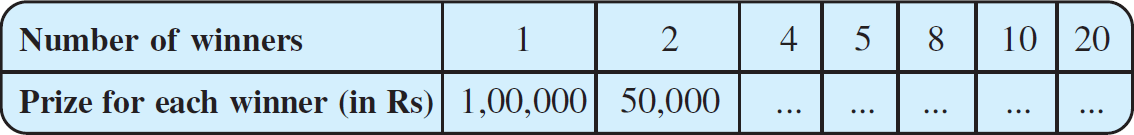As the number of winners increases the prize for each winner decreases that is it is in inverse proportion.
When the number of winners are 4, each winner will get = 100000/4 = ₹25000.
When the number of winners are 5, each winner will get = 100000/5 = ₹20000.
When the number of winners are 8, each winner will get = 100000/8 = ₹12500.
When the number of winners are 10, each winner will get = 100000/10 = ₹10000.
When the number of winners are 20, each winner will get = 100000/20 = ₹5000.

Question 3: Rehman is making a wheel using spokes. He wants to fix equal spokes in such a way that the angles between any pair of consecutive spokes are equal. Help him by completing the following table.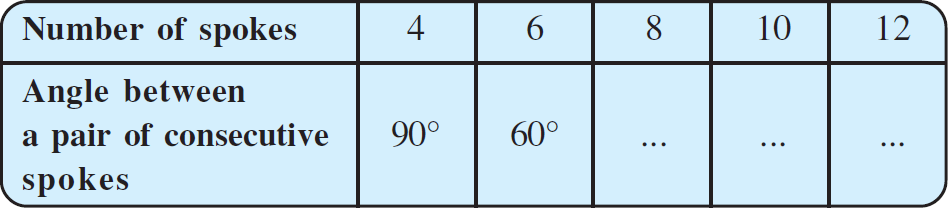i) Are the number of spokes and the angles formed between the pairs of consecutive spokes in inverse proportion?
ii) Calculate the angle between a pair of consecutive spokes on a wheel with 15 spokes.
iii) How many spokes would be needed, if the angle between a pair of consecutive spokes is 40°?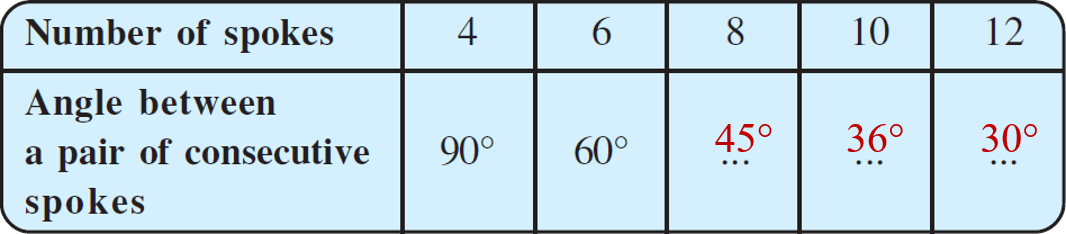As the number of spoke increases the angle between the pair of consecutive spokes decreases that is they are in inverse proportion.
When the number of spokes are 8, angle between a pair of consecutive spokes = 360/8 = 45°.
When the number of spokes are 10, angle between a pair of consecutive spokes = 360/10 = 36°.
When the number of spokes are 12, angle between a pair of consecutive spokes = 360/12 = 30°.

Question 4: If a box of sweets is divided among 24 children, they will get 5 sweets each. How many would each get, if the number of the children is reduced by 4?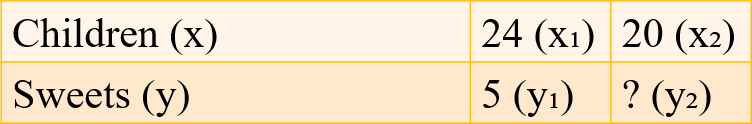= x₁y₁ = x₂y₂
= 24 × 5 = 20 × y₂
= y₂ = 24 × 5/20
= y₂ = 6
Therefore, each children will get 6 sweets, if the number of children is reduced by 4.

Question 5: A farmer has enough food to feed 20 animals in his cattle for 6 days. How long would the food last if there were 10 more animals in his cattle?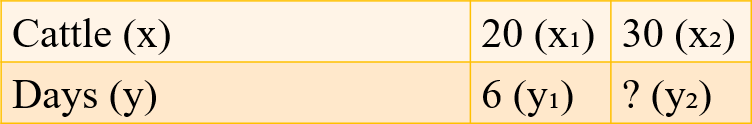= x₁y₁ = x₂y₂
= 20 × 6 = 30 × y₂
= y₂ = 20 × 6/30
= y₂ = 4
Therefore, the food would last for 4 days if there were 10 animals more in his cattle.

Question 6: A contractor estimates that 3 persons could rewire Jasminder’s house in 4 days. If, he uses 4 persons instead of three, how long should they take to complete the job?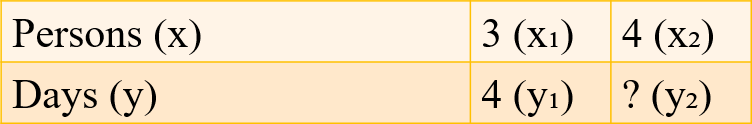= x₁y₁ = x₂y₂
= 3 × 4 = 4 × y₂
= y₂ = 3 × 4/4
= y₂ = 3
Therefore, if the contractor uses 4 persons instead of three, they will take 3 days to complete the job.

Question 7: A batch of bottles were packed in 25 boxes with 12 bottles in each box. If the same batch is packed using 20 bottles in each box, how many boxes would be filled?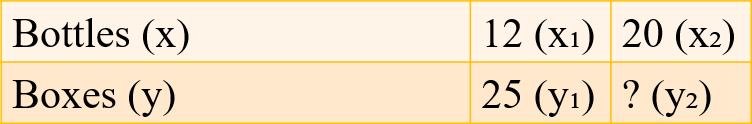= x₁y₁ = x₂y₂
= 12 × 25 = 20 × y₂
= y₂ = 12 × 25/20
= y₂ = 15
Therefore, if the same batch is packed using 20 bottles in each boxes, 15 boxes would be filled.

Question 8: A factory requires 42 machines to produce a given number of articles in 63 days. How many machines would be required to produce the same number of articles in 54 days?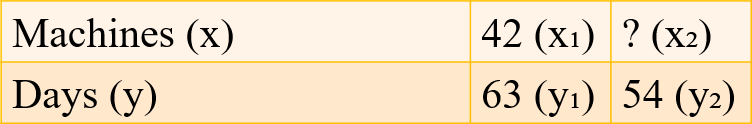= x₁y₁ = x₂y₂
= 42 × 63 = x₂ × 54
= x₂ = 42 × 63/54
= x₂ = 49
Therefore, 49 machines would be required to produce the same number of articles in 54 days.

Question 9: A car takes 2 hours to reach a destination by travelling at the speed of 60 km/h. How long will it take when the car travels at the speed of 80 km/h?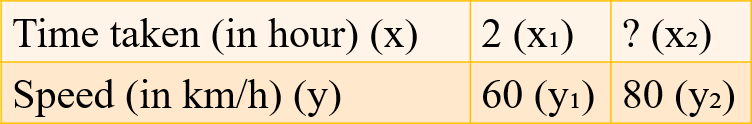= x₁y₁ = x₂y₂
= 2 × 60 = x₂ × 80
= x₂ = 2 × 60/80
= x₂ = 1.5
Therefore, the car can reach the destination in 1.5 hour when the car travels at the speed of 80 km/h.

Question 10: Two persons could fit new windows in a house in 3 days.
i) One of the persons fell ill before the work started. How long would the job take now?
ii) How many persons would be needed to fit the windows in one day?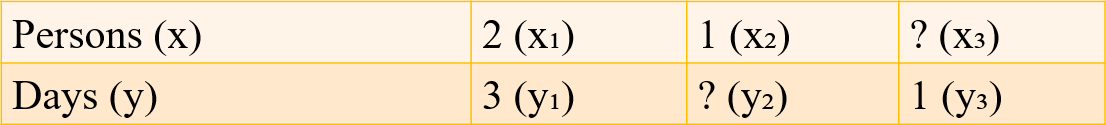i)
= x₁y₁ = x₂y₂
= 2 × 3 = 1 × y₂
= y₂ = 2 × 3/1
= y₂ = 6
Therefore, the time taken to fit new windows in house is 6 days if one of the persons fell ill before the work started.

ii)
= x₁y₁ = x₂y₂
= 2 × 3 = x₃ × 1
= x₃ = 2 × 3/1
= x₃ = 6
Therefore, 6 persons would be needed to fit the windows in one day.

Question 11: A school has 8 periods a day each of 45 minutes duration. How long would each period be, if the school has 9 periods a day, assuming the number of school hours to be the same?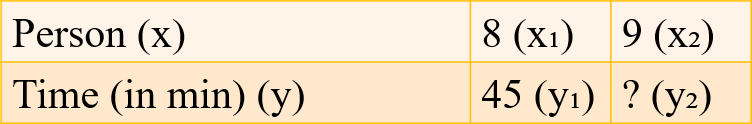= x₁y₁ = x₂y₂
= 8 × 45 = 9 × y₂
= y₂ = 4 × 45/9
= y₂ = 40
Therefore, each period would be 40 minutes, if the school has 9 periods a day, assuming the number of school hours to be the same.